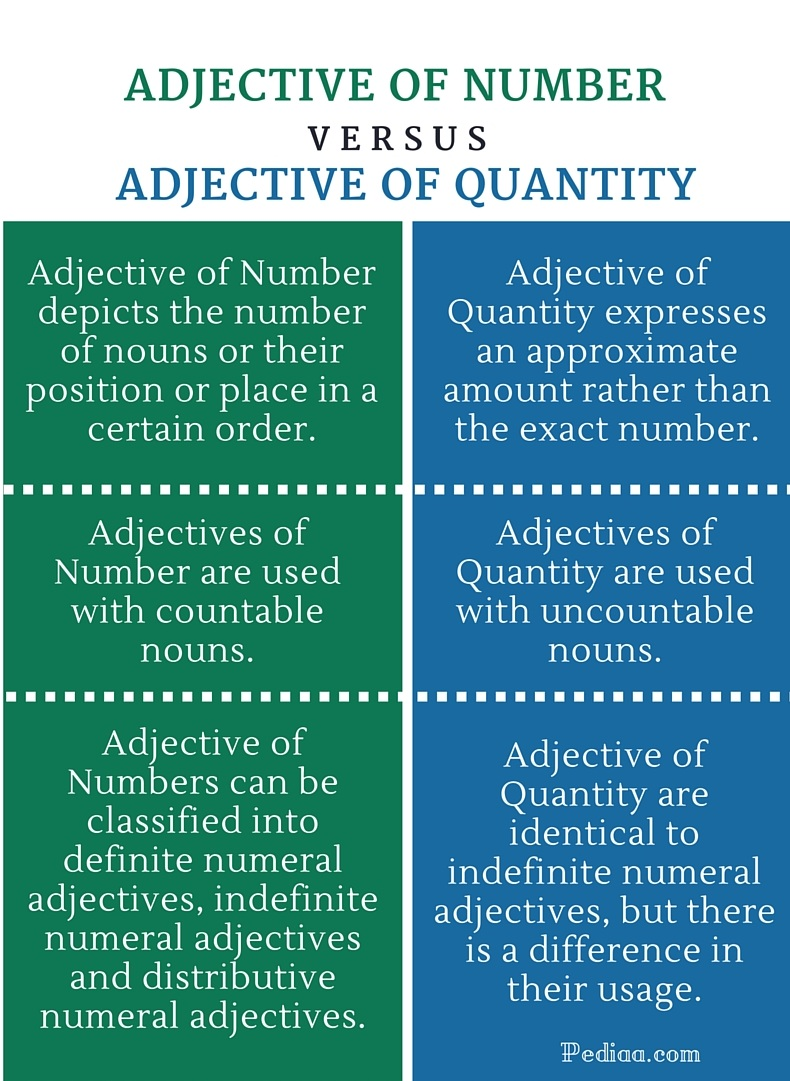# Difference Between Adjective of Number and Quantity

## Main Difference – Adjective of Number vs Quantity

Adjectives of number and adjectives of quantity both indicate an amount. The main difference between adjectives of number and quantity is that adjectives of quantity express an approximate quantity whereas adjectives of number express the exact number.

## What is an Adjective of Number

An adjective of number is an adjective that shows the exact number of persons or things denoted by a noun or pronoun. An adjective of number can also demonstrate a noun’s position or place in a certain order. This is also known as a numeral adjective. Adjectives of number are used with countable nouns.

Definite numeral adjectives denote the exact number of nouns or their exact position. Both cardinal numbers (one, two, three, four, etc.) and ordinal numbers (first, second, third, fourth, etc.) form definite numeral adjectives.

There are thirty students in her class.

I ate five mangoes.

She was the first to join the dance class.

Indefinite Numeral adjectives indicate an approximate amount instead of the exact number. Some, many, few, and several are some examples.

Several students refused to be silent.

Many people attended the meeting.

I bought some eggs.

Distributive numeral adjectives refer to individual nouns within the whole amount. Every, each, either, and another  are some examples of distributive numeral adjectives.

Every student in the class stood up.

Each one of you will get a different project.She picked some wild flowers.

## What is an Adjective of Quantity

An adjective of quantity is an adjective that indicates the quantity. It answers the question how much. Adjectives of quantity are generally used with uncountable nouns. These adjectives express the approximate amount of the nouns rather than the exact number. Many, much, some, several, few, and enough are some examples of adjectives of quantity. The difference between indefinite numeral adjectives and adjectives of quantity is that indefinite numeral adjectives are used with countable nouns whereas adjectives of quantity are used with uncountable nouns. The following sentences will illustrate how they are used in sentences.

I ate some rice and drank some water.

There was little milk at the bottom of the jug.

I had enough exercise for the day.

I don’t have much time; I’ve to leave early.

He has completed most of the work.She drank some water from the fountain.

## Difference Between Adjective of Number and Quantity

### Definition

Adjective of Number depicts the number of nouns or their position or place in a certain order.

Adjective of Quantity expresses an approximate amount rather than the exact number.

### Type of Noun

Adjective of Number is used with countable nouns.

Adjective of Quantity is used with uncountable nouns.

### Classification

Adjective of Quantity are identical to indefinite numeral adjectives, but there is a difference in their usage.